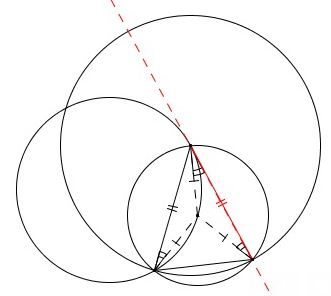# Tangent to Circle in Three Steps

### Problem### Hint

How many points does it take to define a line?

How many circles does it take to define a point?

### Construction

Denote the given point $A.\,$1. Choose an arbitrary point $B\,$ on the circle and draw circle $B(A),\,$ through $A\,$ centered at $B.\,$ Let $D\,$ be the other point of intersection of the two circles.

2. Draw the circle $A(D)\,$ through $D\,$ centered at $A.\,$ Mark point $E,\,$ the second intersection of $B(A)\,$ and $A(D).$

3. Draw the line $AE.$

### Proof 1 of the construction

Let $O\,$ be the center of the given circle.Assume $\angle AOD=2\alpha.\,$ Then $\angle OAD=90^{\circ}-\alpha\,$ and $\angle BAD=\displaystyle \frac{\alpha}{2},\,$ as inscribed angle subtended by half the arc $\overset{\frown}{AD}.$

By the construction, $\Delta ABD=\Delta ABE\,$ such that $\angle BAE=\angle BAD=\displaystyle \frac{\alpha}{2}.\,$ It follows that

\displaystyle\begin{align}\angle OAE&=\angle OAD+\angle BAD+\angle BAE\\ &=(90^{\circ}-\alpha)+2\frac{\alpha}{2}=90^{\circ}. \end{align}

### Proof 2 of the construction

The proof is simply an observation that the angle between the line and a chord equals the inscribed angle opposite the chord, and is therefore tangent.### Acknowledgment

The above problem comes from an uncommon site euclidea, devoted to the problems of Euclidean construction. The site and the problem have been brought to my attention by Konstantin Knop. Proof 2 is by Ted Courant.

### Various Geometric Constructions

• How to Construct Tangents from a Point to a Circle
• How to Construct a Radical Axis
• Constructions Related To An Inaccessible Point
• Inscribing a regular pentagon in a circle - and proving it
• The Many Ways to Construct a Triangle and additional triangle facts
• Easy Construction of Bicentric Quadrilateral
• Easy Construction of Bicentric Quadrilateral II
• Star Construction of Shapes of Constant Width
• Four Construction Problems
• Geometric Construction with the Compass Alone
• Construction of n-gon from the midpoints of its sides
• Short Construction of the Geometric Mean
• Construction of a Polygon from Rotations and their Centers
• Squares Inscribed In a Triangle I
• Construction of a Cyclic Quadrilateral
• Circle of Apollonius
• Six Circles with Concurrent Pairwise Radical Axes
• Trisect Segment: 2 Circles, 4 Lines
• Regular Pentagon Construction by K. Knop
•